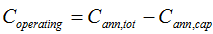# HOMER Grid 1.8The operating cost is the annualized value of all costs and revenues other than initial capital costs.

 Type: Output Variable Units: \$/yr Symbol: Coper,tot

HOMER uses the following equation to calculate the operating cost:where: Cann,tot = the total annualized cost [\$/yr] Cann,cap = the total annualized capital cost [\$/yr]

Tip: The total annualized capital cost is equal to the total initial capital cost multiplied by the capital recovery factor.

HOMER displays the operating cost in the optimization results list, and at the top of the Simulation Results window. You can see the total annualized cost (Total) and the total annualized capital cost (Capital) by clicking the Cost Summary tab in the Simulation Results window and clicking the Annualized radio button under Cost Types.